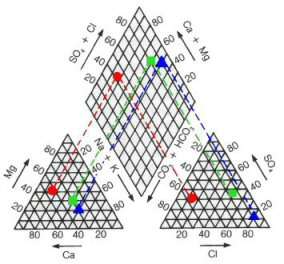Conversion factors for groundwater chemistry

Groundwater chemistry data are usually quoted in milligrams per litre (mg/l). In order to plot these data on one of the graphical methods such as a Piper or Schoeller diagram, it is necessary to convert the units to milli-equivalents (meq/l). Use the conversion factors from the table below and divide the concentration of each ion in mg/l by the factor shown.

For example, a Magnesium concentration of 38 mg/lMg is 38.00 ÷ 12.16 = 3.125 meq/lMg.

 Ion Factor Calcium (Ca) 20.04 Magnesium (Mg) 12.16 Potassium (K) 39.10 Sodium (Na) 23.00 Chloride (Cl) 35.46 Sulphate (SO4) 48.04 Nitrate (NO3) 62.01 Bicarbonate (HCO3) 61.01 Carbonate (CO3) 30.01Determinands may be expressed in different units than the one you want. The following table provides the conversion factors for the most common ones.

 To convert from to multiply by Nitrate as N Nitrate as NO3 0.2258 Nitrite as N Nitrite as NO2 0.3043 Ammonium as N Ammonium as NH4 0.7778 Alkalinity as HCO3 Alkalinity as CaCO3 1.22 Hardness as Ca Hardness as CaCO3 0.40 Sulphate as SO4 Sulphate as SO3 1.193

When you look through old records chemical analyses may be quoted in other units. Frequently 'parts per million' (ppm) are used and these can be treated as being the same as mg/l. Even older records may express concentrations in 'grains per gallon' and can be converted to mg/l by multiplying by 14.25 for imperial gallons or 17.11 for US gallons

This Handy Hint is based on information in my book Field Hydrogeology.